\$B:#>,\$N0lGU(B

\$BKhF|!":#>,\$N0lGU\$r>e\$2\$F\$\$\$^\$9!#(B
\$B:#>,0{\$`\$b\$N\$K:\$\$C\$?\$i!";29M\$K\$7\$F\$_\$F\$/\$@\$5\$\$!#(B

2021\$BG/(B11\$B7n(B

 1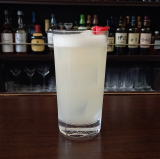(IL^Z/<]8^(B 2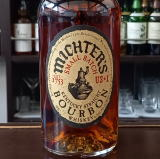(IP8@0=^\$B!!(IJ^0N^](B 3 \$B5Y\$_(B 4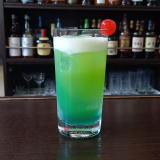(I6XK^1]%12=J^08^(B 5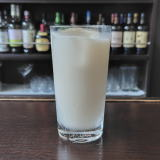(IV08^YD%<^]<^,0(B 6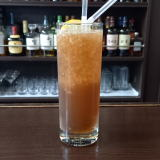(IOXL^%C(0(B 7\$B5Y\$_(B 8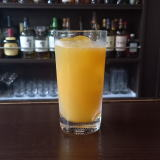(I2@X1]%=8X-0D^W2J^0(B 9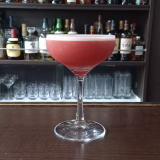(IX=70%LW/<-(B 10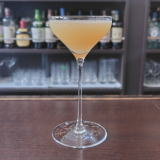(I@^]C^W25](B 11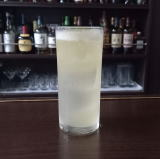(IO[]%L(=^(B 12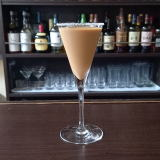(I:^C^(J^%2@X10I(B 13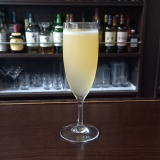(IM_1%;\0(B 14 \$B5Y\$_(B 15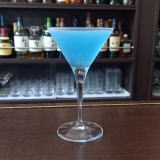(I=@0@^=D%ZK^-0(B 16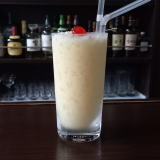(IJ^0<^]%K_F,%:W0@^(B 17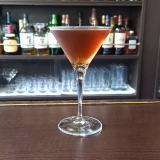(I4RWYD^(B 18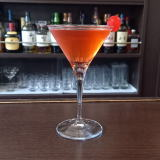(IW]L^W0=^(B 19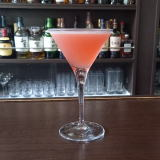(ILW21%@/8(B 20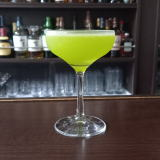(IR[H1(B 22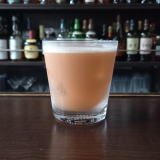(I7=%;^%N^02=^%8^/J^2(B 23 \$B5Y\$_(B 24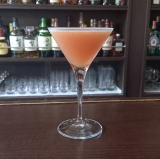(I;^%W=D%D^[/L_(B 25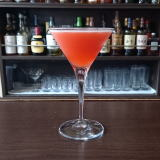(IZC^(%(B80 26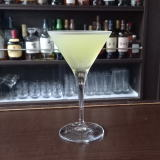(I1YJ^0C(](B 27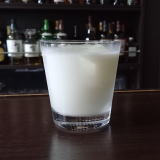(IO[]%PY8(B 28 \$B5Y\$_(B 29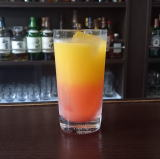(IM_YI%XK^4W(B 30(IZ8^[](B